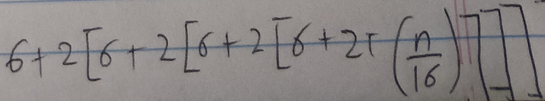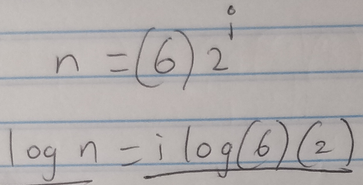# Recurrence relation of a recursive function

Staff member

#### Dr.Peterson

##### Elite Member
Is there any critique about each step?
I think you are trying to find an explicit formula for T by looking for a pattern.

One comment is that I think you need to expand expressions likein order to see the correct pattern.

Another is that these are not equivalent:That probably won't be used in the correct solution, but you do need to learn to be careful with logs.

A thought about the problem itself: Have you considered what happens if n is not a power of 2? I started by calculating T(2), T(3), T(4), and this is instructive. Your final answer is likely to involve something like the greatest-integer function.

One specific question: Can you tell us what steps you were taught to take, so we can understand your approach?

##### New member
I think you are trying to find an explicit formula for T by looking for a pattern.

One comment is that I think you need to expand expressions like
View attachment 28958
in order to see the correct pattern.

Another is that these are not equivalent:
View attachment 28959
That probably won't be used in the correct solution, but you do need to learn to be careful with logs.

A thought about the problem itself: Have you considered what happens if n is not a power of 2? I started by calculating T(2), T(3), T(4), and this is instructive. Your final answer is likely to involve something like the greatest-integer function.

One specific question: Can you tell us what steps you were taught to take, so we can understand your approach?
Step 1: Write the recursive function
Step 2: Expand the recursive function three times
Step 3: Write the last T(n) in terms of i
Step 4: Take the input of T(n) from step 3 and set it to the value of the base case. And solve for i.
Step 5: Plug the value of i in the equation from step 3.

Find c and n when claiming the equation is Big O

##### New member
We do not know the problem statement of your assignment!

Please post the exact problem as it was given to you.
Before I list the 5 steps, the first is a base case and the second is a recursive case of a function,

#### Dr.Peterson

##### Elite Member
You haven't actually quoted the problem statement yet, as requested.
Step 2: Expand the recursive function three times
You haven't expanded, as I use the term. In particular, your step 3 has an expanded form, which doesn't match your results from step 2.
Step 3: Write the last T(n) in terms of i
How is i defined?
Find c and n when claiming the equation is Big O
What does this refer to? Will we understand when you state the problem fully?